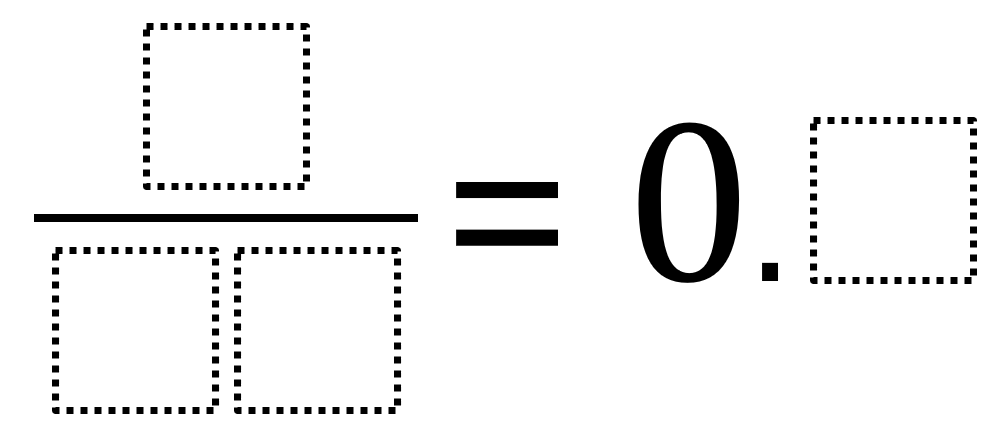Home > Grade 7 > Converting a Fraction to a Decimal

# Converting a Fraction to a Decimal

Directions: Using the digits 1 to 9, at most one time each, place a digit in each box to make a true statement.### Hint

How does choosing the digits for the denominator affect the decimal value?
How might choosing the digit for the decimal make finding the digits for the fraction easier?